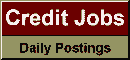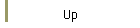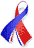DefaultRisk.com the web's biggest credit risk modeling resource.Export citation to:- HTML- Text (plain)- BibTeX- RIS- ReDIF

Alternatives to the Median Absolute Deviation

by Peter J. Rousseeuw of the Universitaire Instelling Antwerpen, and
Christophe Croux of the Universitaire Instelling Antwerpen

December 1993

Abstract: In robust estimation one frequently needs an initial or auxiliary estimate of scale. For this one usually takes the median absolute deviation MAD, = 1.4826 med, { I x, - med,x, 1 }, because it has a simple explicit formula, needs little computation time, and is very robust as witnessed by its bounded influence function and its 5090 breakdown point. But there is still room for improvement in two areas: the fact that MAD, is aimed at symmetric distributions and its low (37%) Gaussian efficiency. In this article we set out to construct explicit and 5090 breakdown scale estimators that are more efficient. We consider the estimator S, = 1.1926 med, {med, I x, - x, ) and the estimator Q, given by the .25 quantile of the distances { I xi - x, ; i <j ) . Note that S, and Q, do not need any location estimate. Both S, and Q, can be computed using O(n log n) time and O(n) storage. The Gaussian efficiency of S, is 5870, whereas Q,, attains 8290. We study S, and Q, by means of their influence functions, their bias curves (for implosion as well as explosion), and their finite-sample performance. Their behavior is also compared at non-Gaussian models, including the negative exponential model where S,, has a lower gross-error sensitivity than the MAD.

Keywords: Bias curve, Breakdown point, Influence function, Robustness, Scale estimation.

Published in: Journal of the American Statistical Association, Vol. 88, No. 424, (December 1993), pp. 1273-1283.

Books Referenced in this Paper:  (what is this?)